# Three classes of graphical matroids

Guest post by Daryl Funk.

Biased graphs, and the frame and lifted-graphic (or simply, lift) matroids associated with them, have been discussed several times already in The Matroid Union blog. Irene Pivotto introduced them in a series of posts, Biased graphs and their matroids I, II, and III. In part II, Irene offered to put money on the truth of the following two conjectures.

Conjecture 1. The class of frame matroids has only a finite number of excluded minors.

Conjecture 2. The class of lift matroids has only a finite number of excluded minors.

If anyone took her up on her offer, you may now collect on your bet. In [CG17], Rong Chen and Jim Geelen exhibit an infinite family of excluded minors for the class of frame matroids, and another for the class of lift matroids. (It is unfair of me to pick on Irene like this — last I heard, bookies were giving 10:1 odds on). These families of excluded minors belong to a third class of matroids having graphical-type structure. So before discussing Rong and Jim’s counter-examples to Conjectures 1 and 2, we had better learn about this new class.

# Quasi-graphic matroids

In his post, Graphical representations of matroids, Jim Geelen discussed a preliminary formulation for a new class of “graphical” matroids, which he there called framework matroids. The goal was to define a single minor-closed class that contains both the classes of frame and lift matroids, and to do so in a way such that (1) the new class maintains or captures the fundamental underlying graphic structure of these matroids, and (2) recognising membership in the new class is tractable — that is, there should be a polynomial-time algorithm to test membership via a rank oracle.

Jim’s goal has largely been realised, with his introduction, along with Bert Gerards and Geoff Whittle, of the class of quasi-graphic matroids, in [GGW17]. There should certainly be a post wholly devoted to this wonderful class of matroids soon. Here, I will tantalise you with just the definition and an example.

Let $M$ be a matroid. A graph $G$ is a framework for $M$ if

1. $E(G)=E(M)$,
2. for each component $H$ of $G$, $r_M(E(H)) \leq |V(H)|$,
3. for each vertex $v$ of $G$, $\operatorname{cl}_M(E(G-v)) \subseteq E(G-v) \cup \{e: e\ \text{is a loop incident to}\ v\},$ and
4. for each circuit $C$ of $M$, the subgraph $G[C]$ induced by $C$ has at most two components.

A matroid is quasi-graphic if it has a framework. The definition is motivated by the following theorem of Paul Seymour, which yields a polynomial-time algorithm to test, via a rank oracle, if a given matroid is graphic. A star of a graph $G$ is the set of edges incident to a vertex $v \in V(G)$ each of whose other endpoint is in $V(G)-v$ (so while $G$ may have loops, loops are not included in any star); $c(G)$ denotes the number of components of $G$.

Theorem 1 [S81]. Let $M$ be a matroid, and let $G$ be a graph. Then $M$ is the cycle matroid of $G$ if and only if

1. $E(G)=E(M)$,
2. $r(M) \leq |V(G)| – c(G)$, and
3. every star of $G$ is a union of cocircuits of $M$.

One can readily see that the requirements for a framework are inspired by the conditions of Theorem 1. One can also see that generalising these conditions to encompass a larger minor-closed class that includes all frame and lift matroids, is not quite straightforward (hopefully, we may learn more about this in a future post). In [GGW17], Jim, Bert, and Geoff show (among other things) that the class of quasi-graphic matroids has the following nice properties:

• It is minor-closed.
• If $(G,\mathcal B)$ is a biased graph, then $G$ is a framework for the lift matroid $LM(G,\mathcal B)$, and $G$ is a framework for the frame matroid $FM(G,\mathcal B)$; thus all lift and all frame matroids are quasi-graphic.
• Given a 3-connected matroid $M$ and a graph $G$, one can check in polynomial time whether $G$ is a framework for $M$.

Jim, Bert, and Geoff conjecture in [GGW17] that there is a polynomial-time algorithm to test, via a rank oracle, if a given matroid is quasi-graphic. In contrast, Rong Chen and Geoff Whittle have recently shown that for each of the classes of frame and lift matroids, testing for membership in the class is intractable [CW16]. More on this in a moment. But first, let us try to get a bit of a feel for what a typical quasi-graphic matroid might look like.

Let us recall some required preliminary concepts. Every frame and every lift matroid may be represented by a biased graph $(G,\mathcal B)$ with $E(G)=E(M)$. For clarity’s sake, I’ll reserve the word circuit for matroids, and use the word cycle for a 2-regular connected subgraph. Recall how the circuits of frame and lift matroids appear in their biased graph representations: they are precisely the edge sets of subdivisions of certain biased subgraphs. Recall, a cycle $C$ of a graph whose edge set is a circuit of the matroid is balanced; otherwise $E(C)$ is independent and $C$ is said to be unbalanced. The figures in Sections 2 and 3 of Irene’s first post on biased graphs, reproduced here for your convenience, illustrate these biased subgraphs.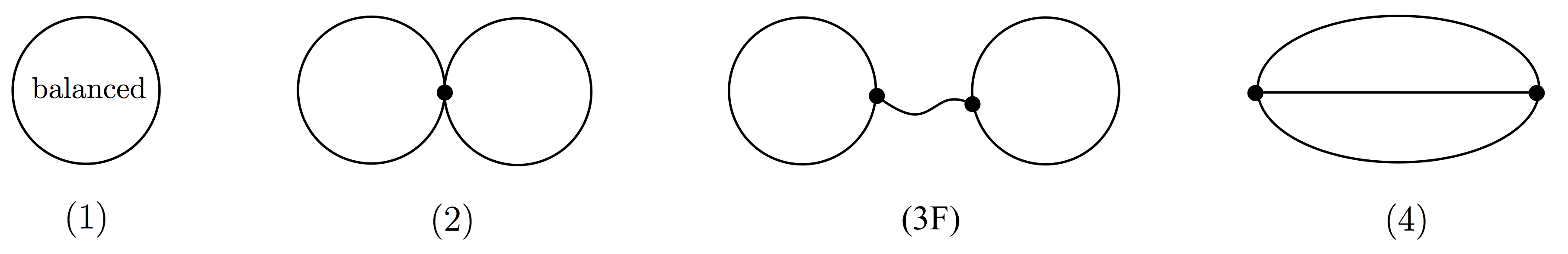Circuits of frame matroids are subdivisions of these graphs. Unlabelled cycles are unbalanced.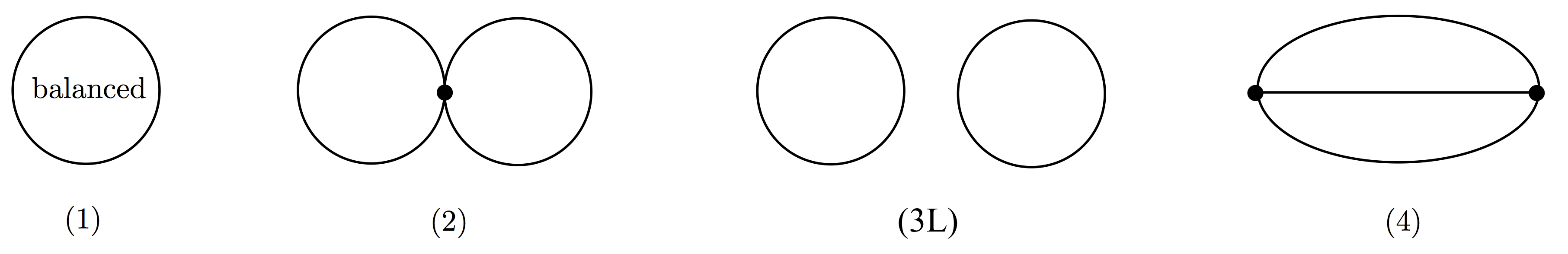Circuits of lift matroids are subdivisions of these graphs. Unlabelled cycles are unbalanced.

Let us call a subdivision of one of these five biased subgraphs (1), (2), (3F), (3L), (4), a circuit-subgraph. Note that the frame and lift matroids associated with a given biased graph differ on just one pair of these circuit-subgraphs, namely, (3F) and (3L) — a pair of vertex disjoint unbalanced cycles forms a circuit of the lift matroid, but is independent in the frame matroid. As Irene has explained in her previous posts, given a biased graph, we get a frame matroid by taking as circuits just circuit-subgraphs of the forms (1), (2), (3F), and (4), we get a lift matroid by taking as circuits just circuit-subgraphs of the forms (1), (2), (3L), and (4), and Tom Zaslavsky has shown that in fact all frame matroids, and all lift matroids, are obtained this way.

What about quasi-graphic matroids? Here is an example. The Vámos matroid (shown below left as a cube, in which the only 4-circuits are the 4 “sides” and just the one “diagonal” plane 2468) is neither a frame matroid, nor a lift matroid, but it is quasi-graphic, with the graph below right providing a framework. (Check that it satisfies the definition!)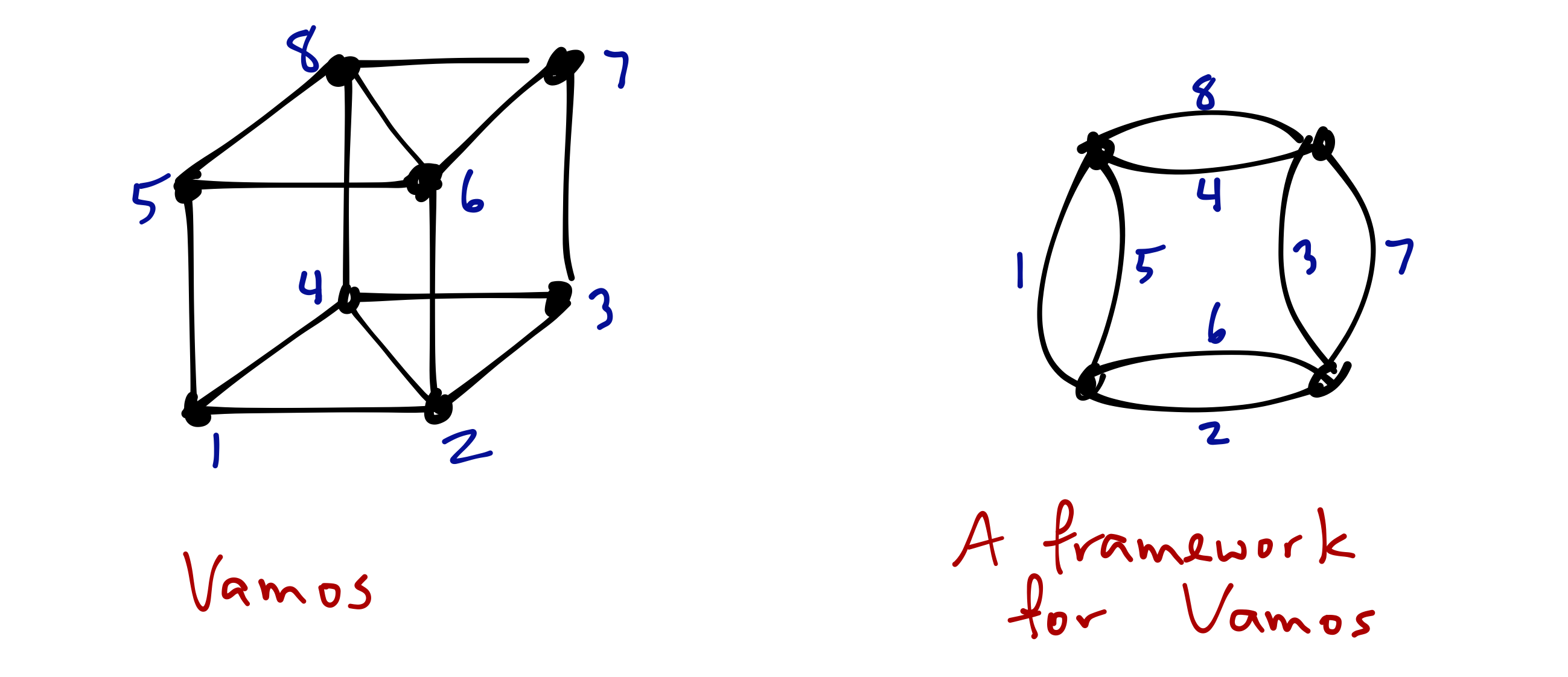Consider the 4-circuits of Vámos, and the subgraphs they form in the framework graph.
The four planes given by the front, back, and sides of the cube each form a circuit-subgraph of type (2), which is a circuit-subgraph for both frame and lift matroids. But together the circuit 2468 and the independent set 1357 prevent this graph from being either a frame or a lift representation for Vámos: circuit 2468 appears as a type (3L) circuit-subgraph, but so does the independent set 1357.

It turns out that (here I’m summarising results of [GGW17]), just as with biased graph representations of frame and lift matroids, the edge set of every cycle in a framework $G$ for a quasi-graphic matroid $M$ is either a circuit of $M$ or is independent in $M$. Further, declaring each cycle of $G$ to be balanced or unbalanced accordingly, just as for frame and lift matroids, yields a biased graph $(G,\mathcal B)$, where $\mathcal B$ denotes the collection of balanced cycles of $G$ (that is, the collection of balanced cycles satisfies the theta property: no theta subgraph contains exactly two balanced cycles). Moreover, every circuit of $M$ appears in $G$ as one of our five circuit-subgraphs (1), (2), (3F), (3L), (4). Conversely, the edge set of each circuit-subgraph of $G$ of one of the forms (1), (2), or (4) is a circuit of $M$, and each of the form of (3F) is either a circuit or contains a circuit of the form (3L).

Thus frameworks for matroids behave very much like biased graph representations for frame and lift matroids. Given a biased graph, taking $\{(1),(2),(3$F$),(4)\}$ as our circuit-subgraphs gives us a frame matroid, taking $\{(1),(2),(3$L$),(4)\}$ as our circuit-subgraphs gives us a lift matroid; and allowing all of $\{(1), (2), (3$F$), (3$L$), (4)\}$ as circuit-subgraphs gives the class of quasi-graphic matroids. This phenomenon is illustrated in the framework for the Vámos matroid above: 2468 is a (3L) circuit-subgraph, while each of the four circuits $1357 \cup e$ for $e \in \{2,4,6,8\}$ are (3F) circuit-subgraphs. Put another way, if a matroid $M$ has a framework having no circuit-subgraphs of type (3F), then we have a biased graph representation for $M$ as a lift matroid; if $M$ has a framework with no circuit-subgraphs of type (3L), then we have a biased graph representation for $M$ as a frame matroid; the Vámos matroid shows that (3F) and (3L) type circuit-subgraphs can coexist in a framework.

# Recognition of frame, and of lift matroids, is intractable

As mentioned above, Rong and Geoff have shown that there can be no algorithm that can determine, via a rank oracle, in time polynomial in the size of the ground set, whether or not a given matroid is a frame matroid. They also show no such algorithm can exist for recognising lift matroids. They do so using two particular families of quasi-graphic matroids, one for frame and one for lift, arising from the same infinite family of framework graphs. More precisely, they prove the following two theorems.

Theorem 2 [CW16]. For any polynomial $p(\cdot)$, there is a frame matroid $M$ such that, for any collection $\mathcal A$ of subsets of $E(M)$ with $|\mathcal A| \leq p(|E(M)|)$, there is a quasi-graphic matroid $N$ that is not frame, such that $E(N)=E(M)$ and for each $A \in \mathcal A$, $r_k(A) = r_M(A)$.

Theorem 3 [CW16]. For any polynomial $p(\cdot)$, there is a lift matroid $M$ such that, for any collection $\mathcal A$ of subsets of $E(M)$ with $|\mathcal A| \leq p(|E(M)|)$, there is a quasi-graphic matroid $N$ that is not lift, such that $E(N)=E(M)$ and for each $A \in \mathcal A$, $r_k(A) = r_M(A)$.

The proofs go like this. Construct an infinite family of biased graphs $(W_k, \emptyset)$. Relaxation of a particular circuit-hyperplane of the lift matroid $LM(W_k,\emptyset)$ yields a quasi-graphic matroid that is no longer lift, but which agrees with $LM(W_k,\emptyset)$ on almost all subsets. Performing the reverse tweak to the frame matroid $FM(W_k, \emptyset)$ yields a quasi-graphic matroid that is no longer frame, but which agrees with $FM(W_k,\emptyset)$ on almost all subsets. The operation reverse to relaxation of a circuit-hyperplane is that of tightening a free basis. A free basis of a matroid is a basis $B$ such that $B \cup e$ is a circuit for each $e \in E(M)-B$. If $B$ is a free basis of $M$, then removing $B$ from the set of bases of $M$ results in a matroid, which we say is obtained by tightening $B$.

Here is the family of biased graphs. For each even integer $k \geq 4$, let $W_k$ be the graph consisting of 4 edge-disjoint $k$-cycles on vertices $u_1, \ldots, u_k, v_1, \ldots, v_k$: one cycle on the $u_i$’s, one cycle on the $v_i$’s (the vertex disjoint pair of blue cycles in the drawing of $W_6$ at below left), and two cycles (red and green in figure) alternating between $u_i$’s and $v_i$’s hitting every second vertex of the blue cycles as shown (below left).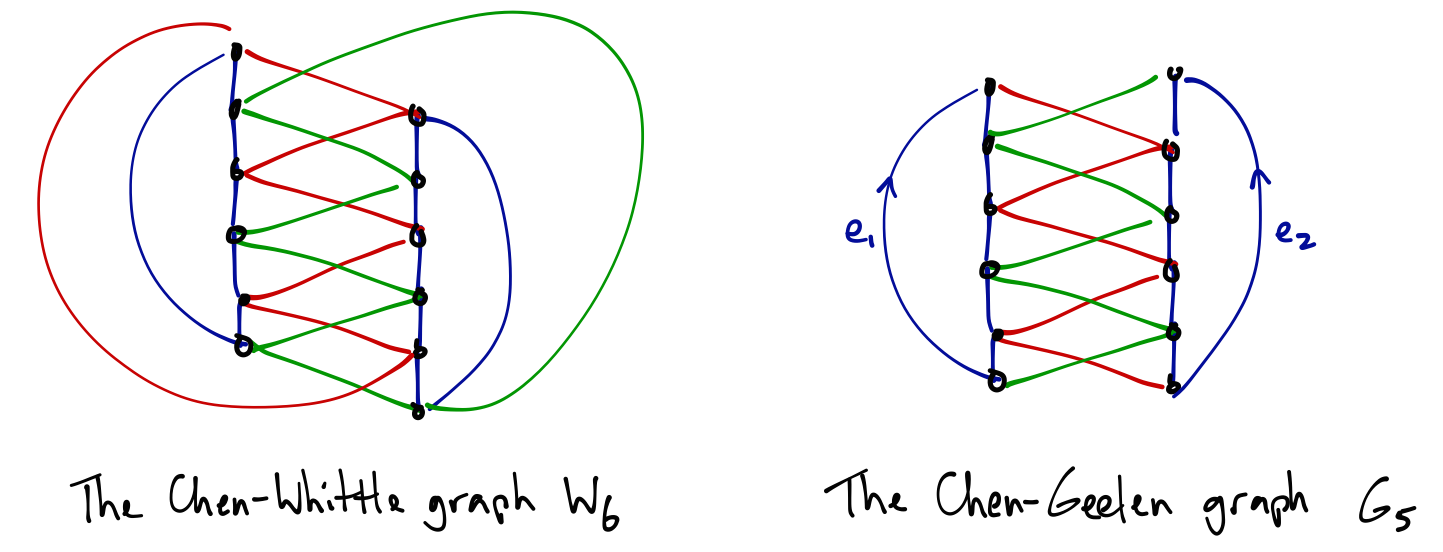Call a green edge and a red edge that cross in this drawing a crossing pair. Observe that $W_k$ has $k$ crossing pairs, and that for every collection $S$ of an even number of crossing pairs, there is a pair of disjoint cycles $C_S^1, C_S^2$ which use precisely these crossing pairs, each of which has length $k$, and which between them traverse all vertices of $W_k$. (See figure below: choosing the 2nd and 4th crossing pair defines the pair of cycles highlighted green and yellow.)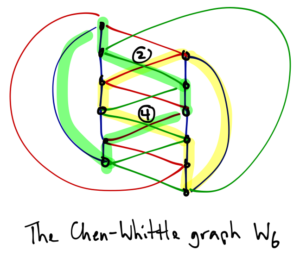The graph $W_k$ has exponentially many collections of crossing pairs: there are $2^{k-1}$ collections consisting of an even number of crossing pairs. Hence $W_k$ has $2^{k-1}$ pairs of disjoint cycles, each pair having the property that together they contain all vertices of $W_k$. Each such pair of cycles is a circuit-hyperplane of the lift matroid $LM(W_k,\emptyset)$, and a free basis of the frame matroid $FM(W_k,\emptyset)$. Let $Z$ be the edge set of a pair of cycles obtained as above from a chosen set of crossing pairs of even cardinality. Let $M_L^Z$ be the matroid obtained from $LM(W_k,\emptyset)$ by relaxing the circuit-hyperplane $Z$. Let $M_F^Z$ be the matroid obtained from $FM(W_k,\emptyset)$ by tightening the free basis $Z$. To distinguish $LM(W_k,\emptyset)$ from $M_L^Z$, and to distinguish $FM(W_k,\emptyset)$ from $M_F^Z$, requires checking the rank of $2^{k-1}$ subsets. This, of course, will be greater than any polynomial in $|E(W_k)|$ for sufficiently large $k$. Since $W_k$ remains a framework for both $M_L^Z$ and $M_F^Z$, both these matroids are quasi-graphic. The proofs are completed by showing that $M_L^Z$ is not a lift matroid, and that $M_F^Z$ is not frame (which takes just another couple of pages in Rong and Geoff’s paper).

The Chen-Whittle graph’s usefulness does not stop here.

# Excluded minors

To disprove Conjectures 1 and 2, Rong and Jim exhibit an infinite family of quasi-graphic matroids, each of which is minor-minimally not frame, and another infinite family of quasi-graphic matroids, each of which is minor-minimally not lifted-graphic. As in Rong and Geoff’s proofs of Theorems 2 and 3, these two families share a common framework. In fact, they again use the Chen-Whittle graphs!

Here is Rong and Jim’s construction. For each odd positive integer $k \geq 5$, let $G_k$ be the graph obtained from the Chen-Whittle graph $W_{k+1}$ defined above by deleting exactly one crossing pair (at right in figure above is shown the Chen-Geelen graph $G_5$). It is convenient to describe the collection of balanced cycles of $G_k$ by group-labelling (group-labelled graphs are described in Irene’s first post on biased graphs, Section 1, 4th bullet point).

For each odd positive integer $k \geq 5$, we obtain a quasi-graphic excluded minor for the class of frame matroids, with framework $G_k$, as follows. Label $G_k$ using the multiplicative group of $\mathbb R$. Referring to the drawing of $G_5$ above: orient each of $e_1$ and $e_2$ “up” from the bottom vertex to the top vertex of the vertical path making up its “side” of the crossing ladder, and assign to both $e_1$ and $e_2$ the label 2. Orient all remaining edges arbitrarily, and assign each label 1. Let $\mathcal B_k$ be the collection of balanced cycles defined by this labelling (that is, ${\mathcal B}_k$ consists of those cycles $C$ for which the product of edge labels, taken while traversing $C$ in a cyclic order, is 1, where we take the multiplicative inverse of each label whose edge is traversed against its orientation). Let $P$ be the paths forming the two vertical sides of the ladder, so $P \cup \{e_1, e_2\}$ is the pair of blue disjoint cycles in the figure, and let $Q$ be the union of the red and green paths. Then $P \cup \{e_1, e_2\}$ and $Q \cup \{e_1, e_2\}$ are free bases of $FM(G_k, \mathcal B_k)$. Let $M_k^F$ be the matroid obtained from $FM(G_k, \mathcal B_k)$ by tightening $P \cup \{e_1, e_2\}$ and $Q \cup \{e_1, e_2\}$. Then $M_k^F/\{e_1, e_2\}$, while quasi-graphic (with framework $G_k/\{e_1,e_2\}$), is an excluded minor for the class of frame matroids.

An excluded minor for the class of lift matroids is obtained in a similar manner. Again orient $e_1$ and $e_2$ up from the bottom to the top vertex of the vertical paths of the ladder. This time, label $G_k$ using elements of the additive group of integers, labelling $e_1$ with $1$, $e_2$ by $-1$, and all remaining edges with $0$. Let $\mathcal B_k$ be the collection of balanced cycles defined by this labelling (that is, $C \in \mathcal B_k$ if and only if when traversing $C$ in a chosen cyclic direction, taking the sum of the labels on its edges, subtracting the label on each edge traversed against its orientation, yields $0$). Let $P$ and $Q$ be as before. Then $P \cup \{e_1,e_2\}$ and $Q \cup \{e_1, e_2\}$ are circuit-hyperplanes of $LM(G_k, \mathcal B_k)$. Let $M_k^L$ be the matroid obtained from $LM(G_k, \mathcal B_k)$ by relaxing $P \cup \{e_1, e_2\}$ and $Q \cup \{e_1, e_2\}$. Then $M_k^L / \{e_1, e_2\}$, while quasi-graphic, is an excluded minor for the class of lift matroids.

Rong and Jim make the following conjecture.

Conjecture 3 [CG17]. The class of quasi-graphic matroids has only a finite number of excluded minors.

Dillon Mayhew and I have recently proved the following three theorems.

Theorem 4 [FM17]. The class of frame matroids has only a finite number of excluded minors of any fixed rank.

Theorem 5 [FM17]. The class of lift matroids has only a finite number of excluded minors of any fixed rank.

Theorem 6 [FM17]. The class of quasi-graphic matroids has only a finite number of excluded minors of any fixed rank.

Rong and Jim’s counterexamples to Conjecture 1 and 2 are both infinite families of excluded minors of ever increasing, arbitrarily large rank (if $G$ is a connected framework for a non-graphic quasi-graphic matroid $M$, then the rank of $M$ is $|V(G)|$). Theorems 4 and 5 say that any such collections of excluded minors for these classes must have this property. Theorem 6 provides evidence toward Conjecture 3 — though no more evidence than Theorems 4 and 5, respectively, provide toward Conjectures 1 and 2! What is perhaps interesting about Theorems 4, 5, and 6 is that — in contrast to what we’ve just seen in the results of Rong, Geoff, and Jim above — the same strategy works for all three classes. Dillon and I set out to prove Theorem 4, and having done so, realised that essentially the same proof also gives us Theorems 5 and 6. Perhaps we can take a look at this strategy in a subsequent post.

# References

[CG17] Rong Chen and Jim Geelen. Infinitly many excluded minors for frame matroids and for lifted-graphic matroids. arXiv:1703.04857

[CW16] Rong Chen and Geoff Whittle. On recognising frame and lifted-graphic matroids. arXiv:1601.01791

[FM17] Daryl Funk and Dillon Mayhew. On excluded minors for classes of graphical matroids. Forthcoming.

[GGW17] Jim Geelen, Bert Gerards, and Geoff Whittle. Quasi-graphic matroids. arXiv:1512.03005

[S81] Paul Seymour. Recognizing graphic matroids. Combinatorica (1981). MR602418

This site uses Akismet to reduce spam. Learn how your comment data is processed.